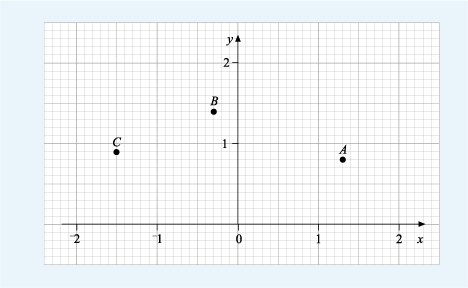Succeed with maths: part 2

Start this free course now. Just create an account and sign in. Enrol and complete the course for a free statement of participation or digital badge if available.

# 2.3 Decimal coordinates

Marking a scale with fractions of whole units allows us to plot points using decimal coordinates such as (1.8, –2.1).

In Figure 9 below, each unit along the axes has been divided into ten, so each grey line represents a tenth of a whole unit, or 0.1. Point A has the coordinates (1.3, 0.8), as it is plotted 1.3 units across to the right of the origin and 0.8 units up.Figure _unit9.2.6 Figure 9 Decimal coordinates

Now, we’ve done the first point for you, complete the coordinates for the other points shown in the next activity.

## Activity _unit9.2.3 Activity 3 Reading decimal coordinates

Timing: Allow approximately 5 minutes

Write down the coordinates for the points B and C on the graph above.

B is plotted at the point which is 3 intervals to the left of the origin on the horizontal scale and 4 intervals past the 1 mark on the vertical scale, i.e. opposite -0.3 on the x-axis and opposite 1.4 on the y-axis. So the coordinates of B are (-0.3, 1.4)

Similarly, the coordinates of C are (-1.5, 0.9).